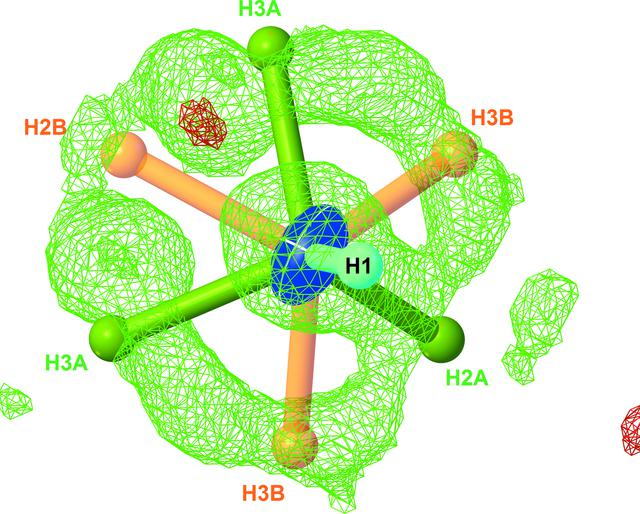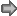disable zoom     view article Figure 2 Difference electron-density map in the vicinity of the N-atom site calculated on the basis of a model including N1 and H1 atoms (R1 = 0.0183, wR2 = 0.0420). The difference map is plotted at the 0.18 e− Å−3 level with a resolution of 0.05 Å (Dolomanov et al., 2009). At this level, only positive residuals are observed (green wire), corresponding essentially to the N—H σ bond and missing H atoms. Positions for all H atoms in the final refinement (R1 = 0.0163, wR2 = 0.0296) are superimposed on the calculated difference map, showing the fit between the experimental data and the proposed model.IUCrDATA
ISSN: 2414-3146